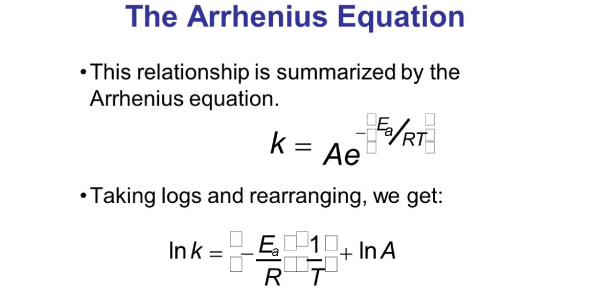# What Do You Know About Arrhenius Equation?

Approved & Edited by ProProfs Editorial Team
The editorial team at ProProfs Quizzes consists of a select group of subject experts, trivia writers, and quiz masters who have authored over 10,000 quizzes taken by more than 100 million users. This team includes our in-house seasoned quiz moderators and subject matter experts. Our editorial experts, spread across the world, are rigorously trained using our comprehensive guidelines to ensure that you receive the highest quality quizzes.
A
Community Contributor
Quizzes Created: 145 | Total Attempts: 38,511
Questions: 10 | Attempts: 393SettingsIn the late 1880s, Nobel Prize-winning Swedish scientist Arrhenius proposed Arrhenius equation, which is a formula for the temperature dependence of reaction rates.
Presently, it is an empirical relationship that can be used to model the temperature variation of diffusion coefficients, the population of crystal vacancies, creep rates, and many other thermally-induced processes and reactions.

• 1.

### What is the formula for Arrhenius equation?

• A.

K=A*exp (-E a*R*T)

• B.

K=A*exp (-E a/T*R)

• C.

K=A*exp (-E a/R*T)

• D.

K=A*exp (E a/R*T)

C. K=A*exp (-E a/R*T)
Explanation
The Arrhenius equation is used to describe the temperature dependence of reaction rates. It states that the rate constant (k) of a reaction is equal to the pre-exponential factor (A) multiplied by the exponential term, which is given by exp (-Ea/RT), where Ea is the activation energy, R is the gas constant, and T is the temperature in Kelvin. Therefore, the correct formula for the Arrhenius equation is k = A*exp (-Ea/RT).

Rate this question:

• 2.

### What does k represent?

• A.

Rate coefficient

• B.

Kepler rate

• C.

Rate of the equation

• D.

Kelvin value

A. Rate coefficient
Explanation
The correct answer is "Rate coefficient". In chemical kinetics, the rate coefficient (k) represents the proportionality constant between the rate of a reaction and the concentrations of the reactants. It determines the speed at which a reaction takes place and is specific to each reaction. The rate coefficient is determined experimentally and is influenced by factors such as temperature, pressure, and catalysts.

Rate this question:

• 3.

### What does A represent?

• A.

Arrhenius value

• B.

Archimedes principle

• C.

Constant

• D.

Area

C. Constant
Explanation
The correct answer is "Constant." A represents a constant value, which means it does not change. It is not related to Arrhenius value, Archimedes principle, or area. A constant can be used in various mathematical equations and formulas to represent a fixed value that remains unchanged throughout the calculation.

Rate this question:

• 4.

### What does Ea represent?

• A.

Activation energy

• B.

Constant

• C.

Arrhenius value

• D.

Energy

A. Activation energy
Explanation
Ea represents activation energy, which is the minimum amount of energy required for a chemical reaction to occur. It is the energy barrier that must be overcome for reactant molecules to transform into products. Activation energy determines the rate at which a reaction proceeds, with higher activation energy leading to slower reactions. This term is commonly used in the context of the Arrhenius equation, which relates the rate constant of a reaction to the activation energy and temperature.

Rate this question:

• 5.

### What does R represent?

• A.

Robert value

• B.

Pressure

• C.

Universal gas constant

• D.

Area

C. Universal gas constant
Explanation
R represents the Universal gas constant. This constant is used in the ideal gas law equation to relate the pressure, volume, and temperature of a gas. It is denoted by the symbol R and has a value of 8.314 J/(mol·K). The Universal gas constant is an important constant in thermodynamics and is used in various calculations involving gases.

Rate this question:

• 6.

### What does T represent?

• A.

Time

• B.

Temperature

• C.

Trigonometric function

• D.

T is a constant

B. Temperature
Explanation
T represents temperature.

Rate this question:

• 7.

### What is the value of R?

• A.

8.314 x 10^-1 kJmol^-1K^-1

• B.

8.314 x 10^-2 kJmol^-1K^-1

• C.

8.314 x 10^-3 kJmol^-1K^-1

• D.

8.314 x 10^-4 kJmol^-1K^-1

C. 8.314 x 10^-3 kJmol^-1K^-1
Explanation
The value of R is 8.314 x 10^-3 kJmol^-1K^-1.

Rate this question:

• 8.

### In logarithmic form, how is the equation written?

• A.

Ln k = ln A - Ea/RT

• B.

Ln k = ln A - Ea*RT

• C.

Ln A = ln k - Ea/RT

• D.

Ln A = ln k - Ea*RT

A. Ln k = ln A - Ea/RT
Explanation
The equation is written in logarithmic form as ln k = ln A - Ea/RT. This form represents the natural logarithm of the rate constant (ln k) being equal to the natural logarithm of the pre-exponential factor (ln A) minus the activation energy (Ea) divided by the gas constant (R) multiplied by the temperature (T).

Rate this question:

• 9.

### Who proposed the equation?

• A.

Steven Arrhenius

• B.

Svante Arrhenius

• C.

Steph Arrhenius

• D.

Pat Arrhenius

B. Svante Arrhenius
Explanation
Svante Arrhenius proposed the equation.

Rate this question:

• 10.

### When was the equation proposed?

• A.

1888

• B.

1889

• C.

1990

• D.

1991

B. 1889
Explanation
The equation was proposed in 1889.

Rate this question:

Related TopicsBack to top